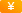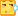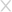## 机器学习入门之像使用Print一样使用算法检测WebShell

2018-12-11180325人围观 ，发现 6 个不明物体

*本文原创作者：littt0，本文属FreeBuf原创奖励计划，未经许可禁止转载

## 0×00 pre

https://github.com/zltningx/webshellgg

## 0×01 准备样本

https://github.com/tennc/webshell

https://github.com/JohnTroony/php-webshells

https://github.com/ysrc/webshell-sample

https://github.com/tanjiti/webshellSample

https://github.com/xl7dev/WebShell

https://github.com/WordPress/WordPress

https://github.com/typecho/typecho

https://github.com/bcit-ci/CodeIgniter

https://github.com/laravel/laravel

https://github.com/symfony/symfony

## 0×02 实现

``pip install sklearn``

### step1： 特征提取

``def load_file(file_path): t = b'' with open(file_path, "rb") as f: for line in f: line = line.strip(b'\r\n') t += line return t``

``def load_files(path): files_list = [] for r, d, files in os.walk(path): for file in files: if file.endswith('.php'): #过滤非php文件 file_path = r + '/' + file print("Load {}".format(file_path)) t = load_file(file_path) files_list.append(t) return files_list``

``webshell_files = load_files(webshell_dir)``

``wp_files = load_files(normal_dir)``

``black_count = len(webshell_files)white_count = len(wp_files)``

``y1 = *black_county2 = *white_count``

``x = webshell_files + wp_filesy = y1 + y2``

``cv = CountVectorizer(ngram_range=(2, 2), decode_error="ignore", token_pattern=r'\b\w+\b', min_df=1, max_df=1.0, max_features=max_features)x = cv.fit_transform(x).toarray()``

``transformer = TfidfTransformer(smooth_idf=False)x = transformer.fit_transform(x).toarray()``

### step2： MLP算法

``clf = MLPClassifier(solver="lbfgs", alpha=1e-5, hidden_layer_sizes=(5, 2), random_state=1)``

``x_train, x_test, y_train, y_test = train_test_split(x, y, test_size=0.4, random_state=0)clf.fit(x_train, y_train)``

``print(np.mean(y_test==clf.predict(x_test)))``### step3： 编写检测函数

``def check(clf, cv, transformer, path): all = 0 webshell = 0 not_webshell = 0 for r, d, files in os.walk(path): for file in files: file_path = r + '/' + file t = load_file(file_path) t_list = list() t_list.append(t) x = cv.transform(t_list).toarray() x = transformer.transform(x).toarray() y_pred = clf.predict(x) all += 1 if y_pred == 1: print("{} is webshell".format(file_path)) webshell += 1 else: not_webshell += 1 print("全部文件：{}，webshell {} 未检出：{} 检出率：{}".format(all, webshell, not_webshell, webshell/all))``

``x = cv.transform(t_list).toarray()x = transformer.transform(x).toarray()``### step4: 持久化

``from sklearn.externals import joblib``

``import pickle``

MLPClassifier对象

CountVectorizer对象

TfidfTransformer对象

``joblib.dump(clf, "mlp.pkl")joblib.dump(cv, "cv.pkl")joblib.dump(transformer, "transformer.pkl")``

``joblib.load("mlp.pkl")``

## 0×03 END

*本文原创作者：littt0，本文属FreeBuf原创奖励计划，未经许可禁止转载### 已有 6 条评论

• (5级)  2018-12-11 回复 1楼

666

• (2级) 这家伙可能不懒，其实就是不想写个人描述！  2018-12-11 回复 2楼

学习学习

• (1级) 本人没有脸  2018-12-11 回复 3楼

当训练数据一大，比如到上T级别了，精度很好了，那是不是程序运行的效率又下降呢

•helloworld  2018-12-11 回复 4楼

一波操作猛如虎，一看战绩0-5。
数据集太少，缺少一些变异。
max_features 25000还是少了点，维度有限的情况下，考虑做数据预处理。
试试其它算法，说不定准确率更高。

• (1级)  2019-07-23 回复 5楼

github.com 上的代码clone回来跑，结果和文章不对应。。
0.5412322274881517
metrics.accuracy_score:
0.5412322274881517
metrics.confusion_matrix:
[[1142 0]
[ 968 0]]
metrics.precision_score:
0.0
metrics.recall_score:
0.0
metrics.f1_score:
0.0

• (1级)  2019-07-23 回复

@ damage  复现了，把max_features改大了，导致结果反而差了### 相关阅读### 填写个人信息

• 可以给我们打个分吗？